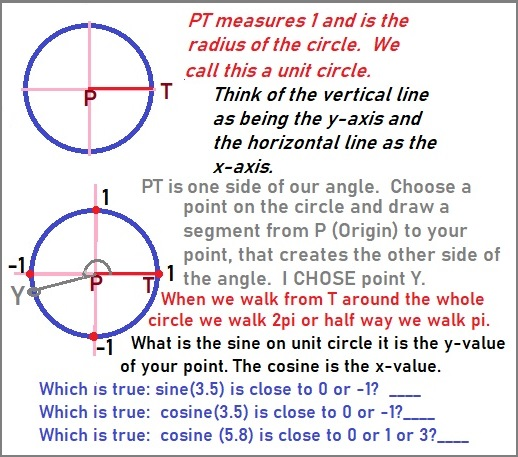# Lockdown math episode 2-part 1: Using the Unit circle of Trig

Posted on: April 22, 2020 Posted by: Susan Johnsey Comments: 1Tuesday April 21, 2020, The Youtube channel 3Blue1Brown streamed a lesson using the unit circle to derive the half-angle formula of trigonometry. He used several concepts in trig, but not in the usual order! In this short lesson I will present a couple of these and then you should look for my next lesson for more of the topics.

Next week they plan to relate it to complex numbers. Did you know complex numbers, (numbers using the i) related to sine and cosine of trig! How you say, well the presentation does take a few skills from Algebra and the trig functions. I will start with the unit circle. What is that? It is a circle whose radius is 1. That means if you walk from the center to the circle then you walked 1 meter or 1 foot or 1 inch or 1 mm or ? Get the idea?

So what if now you walked around the entire circle. How far did you walk? The radius is 1 so the diameter is 2 and the circumference is 2pi. Hope you recall the formula for the circumference ( walking all around the circle) is pi times d . or pi times 2 times radius Either way for the unit circle if you walk all the way around then you walked 2p distance. HOW FAR DO YOU WALK IF YOU WALK HALF-WAY AROUND THE CIRCLE (recall its radius is 1). What is half of 2pi? It is 1pi. What if we don’t even walk half, but instead walk half of half ( that is 1-fourth) of the circle? That would be half of the 1pi or 1pi/2. Excellent. NOW ONE MORE THING about the UNIT CIRCLE. Recall the pi (Greek letter) represents the number pi that we use for crcles. It is a number that is irrational ( goes on for ever). It is 3.1415 and so on. We round it to 3.14 in high school.

So what is 3 units around the circle? That number, 3, is less than pi so it means we walked a bit less than half way around the circle. In the video after he used the unit circle he explained the sine and cosine using unit circle. If that was a bit hard (watching the circle and the wavy curve) just know that he was calling the point on the circle where you had walked to a special name rather than the usual name. What is the usual? Of course you know points are a pair of 2 numbers and the first is x and the second is y. We write them (x, y) but in the video he was showing you the wavy graph and the circle side by side and he was looking for the x and y value for each of his orange points. and using that to create the wavy graph Main thing to know: the point on the circle has x and y values but he called the x: the cosine of the angle and he called y: the sine of the angle. What angle! Angles have 2 sides. Draw a line segment from your point on the circle to the Origin (0, 0). MAke it dark and then draw a segment on the x-axis starting at the ORIGIN and stopping when you touch the circle. Now look again at the angle created by those two segments.

Did you get the quiz questions right?

• Which is true: sine(3) is close to 0 or -1? ____
• Which is true: cosine(3) is close to 0 or -1?____
• Which is true: cosine (6) is close to 0 or 1 or 3?_____
• Read my prior paragraph if you are not sure as you just need to know approximately where the 3 and 6 are on the circumference of the unit circle, LOOK AT THE GRAPH. Mark a point on the circle for 3 and for 6. Then you will know by thinking about the x-value of the POINT as being cosine of the angle or thinking about the y-value of the POINT as being the sine of the angle. Did you get the questions?

Another topic he mentioned about the angle is that sometimes we use degrees to measure angles. But that is an old method and we do stop using it in higher math. You have studied degrees in middle school. Just know that pi (half way around the circle) means the angle is a straight angle and the measure is 180 degrees. So that means the pi measurement for the angle is the same as 180 degrees. The new way of measuring does have a name. It is radians (it is based off the radius of the unit circle). WRITE THIS down: pi radians is equal to 180 degrees. Answer these questions.

• Thus what does pi/2 radians =_____degrees.
• What does pi/3 radians= ______degree.
• What does pi/4 radians= _______degrees.
• What does 2pi/3 radians= ______degrees; hint double you second blank.
• What does 3pi/4 radians =______degrees.

### 1 people reacted on this

1.נערות ליווי says:

Itís hard to find knowledgeable people for this topic, but you seem like you know what youíre talking about! Thanks